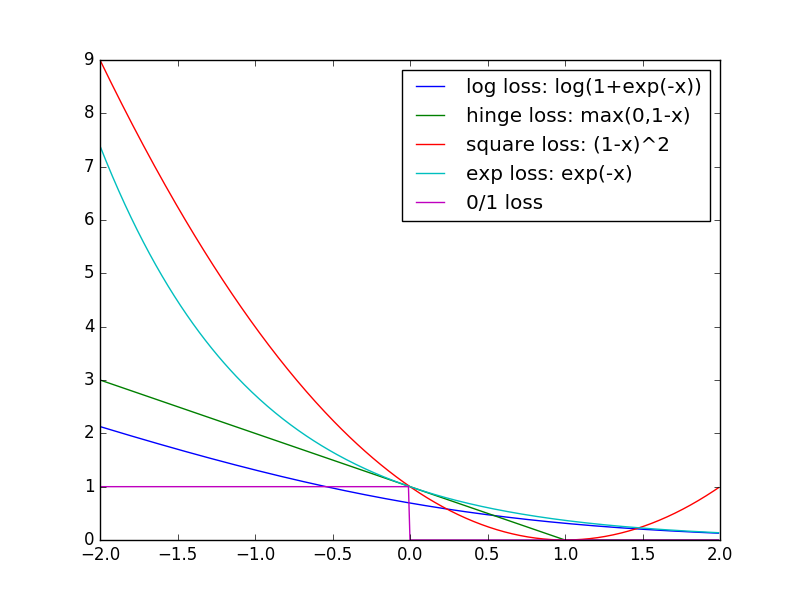# {*no-status title-slide custom-title} // comment- Author: - Supervisors: Rémi Emonet and Marc Sebban - Date:
## Supervised Learning by Risk Minimization
$h^* = \mathrm{arg}\min_{h \in \mathcal{H}} \:\: \frac{1}{m}\sum_{i=1}^m 1_{y_i \neq h(x_i)}$ $\mathcal{S} = [(x_i,y_i)]_{i=1}^m \sim P = X \times Y$0-1 loss
## Surrogate Losses## Bregman Divergence
For any function $\phi : C \to \mathbb{R}$ such that: - $\phi$ is continuously differentiable - $\phi$ is strictly convex - $C$ is a convex set the Bregman Divergence associated to $\phi$ between $x, {x}' \in C$ is
$D_{\phi}(x,{x}') = \phi(x)-\phi({x}')-(x-{x}') \nabla_{\phi}({x}')$
## Squared Euclidean Distance
$\left ||x - {x}' |\right|^2 = (x-{x}')*(x-{x}')$

$\phi = \left ||x |\right|^2 = x*x$ $D_{\phi}(x,{x}') = \left ||x |\right|^2 - \left ||{x}' |\right|^2 - (x-{x}')*2 {x}'$ $\:\:\:\:\:= \left ||x |\right|^2 - 2x*{x}' + \left ||{x}' |\right|^2 = \left ||x - {x}' |\right|^2$ ## Statement
"Any distance that for any distribution of points the mean point minimizes the average distance to all the others is a Bregman Divergence."

Banerjee, Arindam, Xin Guo, and Hui Wang. "On the optimality of conditional expectation as a Bregman predictor." Information Theory, IEEE Transactions on 51.7 (2005): 2664-2669.
## Other examples

### Mahalanobis Distance

$\phi(x) = x^T A x$ $D_{\phi}(x,{x}') = (x-{x}')^T A (x-{x}')$

### Kullback-Leibler Divergence

$\phi(x) = \sum_{j=1}^n x_j \log x_j$ $D_{\phi}(x,{x}') = \sum_{j=1}^n x_j \log \frac{x_j}{{x}'_j}$ ## Properties
$\forall x,{x}'$ in $C$ and $\lambda \geq 0$: 1. $D_{\phi}(x,{x}') \geq 0$ 2. Convexity in $x$ 3. $D_{\phi_1+\lambda \phi_2}(x,{x}') = D_{\phi_1}(x,{x}') +\lambda D_{\phi_2}(x,{x}')$ ## Back to Surrogate Losses Most of the margin-based losses $F_{\phi}$ can be rewritten as: $F_{\phi}(yh(x)) = D_{\phi}(y^* ,\nabla_{\phi}^{-1}(h(x))) = \phi^*(-yh(x))$ where * $y^* \in 0,1$ * $y \in -1,1$ * $\nabla^{-1}$ is the inverse of the gradient * $\phi$ is permissible * $\phi^*(a) = \sup_{x} (ax-\phi(x))$ ## Legendre Conjugate
$\phi^*(a) = \sup_{x} (ax-\phi(x))$
## Example: Logistic Loss
$\phi(x) = x \log x + (1-x) \log (1-x)$ $F_{\phi}(x) = \log(1 + \exp(-x))$
## An Example of Application for Weakly Labeled Data
$F_{\phi}(yh(x)) = \frac{\phi^*(-yh(x)) - a_{\phi}}{b_{\phi}}$
$R_{\phi}(X,Y,h) = \frac{b_{\phi}}{m} \sum_{i=1}^{m} \sum_{\sigma \in -1,1} \beta_i^{\sigma} F_{\phi}(\sigma h(x_i))$ $\:\:\:\:\:\:\:\: - \frac{1}{m} \sum_i \beta_i^{-y_i} y_i h(x_i)$

/ automatically replaced by the authorautomatically replaced by the title